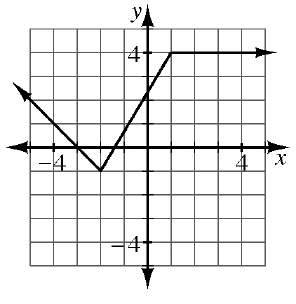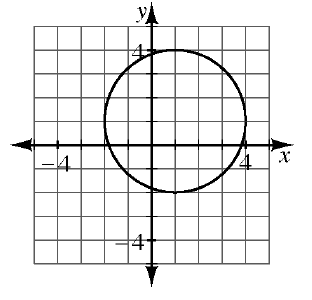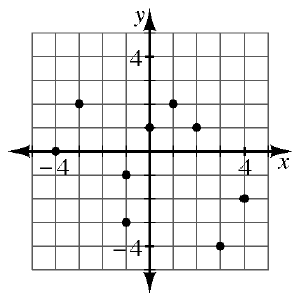### Home > CCAA8 > Chapter 11 Unit 12 > Lesson CCA: 11.3.3 > Problem11-99

11-99.

The graphs of several relations are shown below. Decide if each is a function. If the relation is not a function, explain why not.

1.A relation is only a function if there is only one $y$-value for each $x$-value. Are there any $x$-values on this graph that have multiple $y$-values?

This relation is a function.

1.See the help for part (a).

This relation is not a function. Be sure to explain why.

1.See the help for part (a).